# segclust2d

This guide will organize around 5 sections : - A first section introducing the package and giving basic instruction for installation. - Second, basic examples about how to use the segmentation or segmentation/clustering functions - Then we will explore diverse tools available to explore the outputs of the segmentation. - We will also explore advanced options such as the different input data types available or the possibility of subsampling - Finally we will see additional tools allowing for covariate calculations, such as smoothed speed or turning angle at constant step length.

## Introduction

segclust2d provides R code for two methods of segmentation and joint segmentation/clustering of bivariate time-series. It was originally intended for ecological segmentation (home-range and behavioural modes) but can be easily applied on other type of time-series. The package also provides tools for analysing outputs from R packages moveHMM and marcher.

## Installation

For the CRAN version :

install.packages("segclust2d")

If you want the newest version, you can install segclust2d from github with:

devtools::install_github("rpatin/segclust2d")

# Examples

The algorithm can perform a segmentation of the time-serie into homogeneous segments. A typical case is the identification of home-range behaviour. It can also perform an integrated classification of those segments into clusters of homogeneous behaviour through a segmentation/clustering algorithm. This is generally used to identify behavioural modes. Input data can be a data.frame (shown in the first examples), a Move object or a ltraj object (from package adehabitatLT), both shown in section Other data types

## Segmentation

library(segclust2d)
data(simulshift)

simulshift is an example dataset containing a simulation of home-range behaviour with two shifts. It is a data.frame with two columns for coordinates : x and y. We can now run a simple segmentation with this dataset to find the different home-ranges. You can specify the variables to be segmented using argument seg.var. The function allow rescaling of variable (not recommended for segmentation on coordinates), with argument scale.variable.

The segmentation require arguments lmin, the minimum length of a segment and Kmax, the maximum number of segments. By default Kmax will be set to 0.75*floor(n/lmin), with n the number of observations. However this can considerably slow the calculations so do not hesitate to reduce it to a reasonable value. Be carefull if you want to fix a higher Kmax the algorithm tend to over-segment (which you can check by looking at the segmentation or the likelihood curve)

shift_seg <- segmentation(simulshift, lmin = 300, Kmax = 25, seg.var = c("x","y"), subsample_by = 60, scale.variable = FALSE)
#> Segmentation on x and y

Segmentation is performed through a Dynamic Programming algorithm that finds the best segmentation given a number of segment. For each number of segment, the optimal segmentation is associated with a likelihood value. By default, the algorithm choose the number of segment given a criterium developped by Marc Lavielle based on the value of the second derivative of the penalized likelihood. This criterium use a threshold value of S = 0.75, but a different threshold can be specified. Argument subsample_by controls subsampling and will be explored in section subsampling

segmentation() returns an object of segmentation-class for which several methods are available (see section exploring outputs). The most important one is plot.segmentation, that shows the segmented time-series.

plot(shift_seg)
#> Lavielle-selected number of segment : 3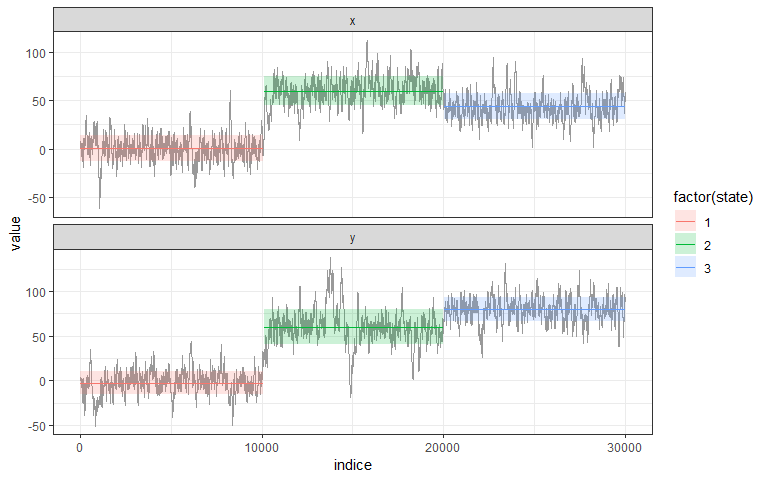By default, plot.segmentation shows the best segmentation, but one can specify a given number of segments (inside the range 1:Kmax). See segmentation-class for additional informations.

plot(shift_seg, nseg = 10)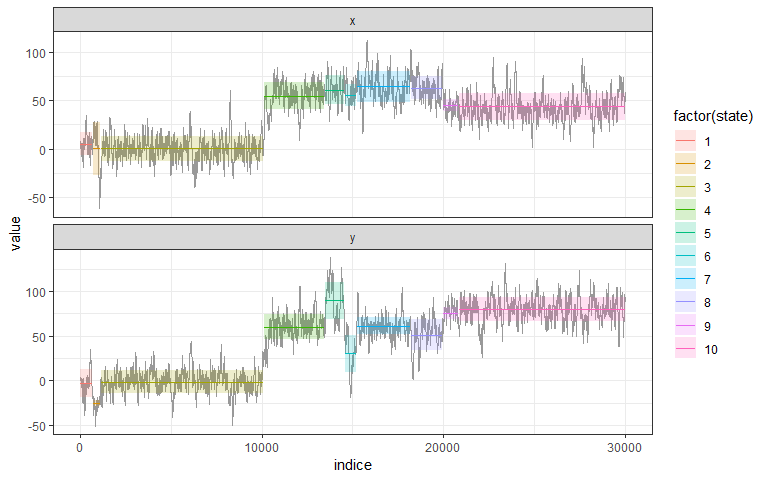The second important method is plot_likelihood that shows the log-likelihood of the best segmentation versus the number of segments and highlights the one chosen with Lavielle’s criterium. The likelihood should show an increasing curve with a clear breakpoints for the optimal number of segment. Note that with real data breaks are often less clear than for that example. An artifactual decrease of likelihood can happen for large number of segment when Kmax is too high (close to n/lmin) and correspond generally to an oversegmentation.

plot_likelihood(shift_seg)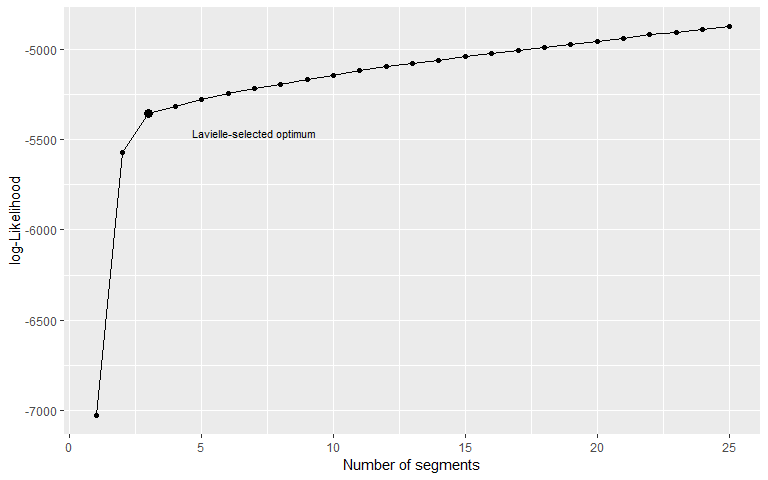## Segmentation-Clustering

data(simulmode)
simulmode$abs_spatial_angle <- abs(simulmode$spatial_angle)
simulmode <- simulmode[!is.na(simulmode\$abs_spatial_angle), ]

simulmode is an example dataset containing a movement simulation with three different movement mode. It is a data.frame with 11 columns, with coordinates and several covariates. Be careful to check your dataset for missing value.

We can now run a joint segmentation/clustering on this dataset to identify the different behavioural modes. As in segmentation, you can specify the variables to be segmented using argument seg.var. The function allow rescaling of variable (recommended for segmentation/clustering to identify behavioural state), with argument scale.variable.

For a joint segmentation/clustering one has to specify arguments lmin, the minimum length of a segment and Kmax, the maximum number of segments, and ncluster a vector of number of class. By default Kmax will be set to 0.75*floor(n/lmin), with n the number of observations. Be carefull if you want to fix a higher Kmax the algorithm tend to over-segment (which you can check by looking at the segmentation or the likelihood curve)

mode_segclust <- segclust(simulmode, Kmax = 20, lmin=10, ncluster = c(2,3), seg.var = c("speed","abs_spatial_angle"), scale.variable = TRUE)
#> Segmenting - 2 class
#> Smoothing  - 2 class
#> Segmenting - 3 class
#> Smoothing  - 3 class

segclust() returns also an object of segmentation-class for which the same methods are available (see section exploring outputs). The most important one is again plot.segmentation, that shows the segmented time-series.

plot(mode_segclust)
#> BIC-selected number of class : 3 class.
#> BIC-selected number of segment : 15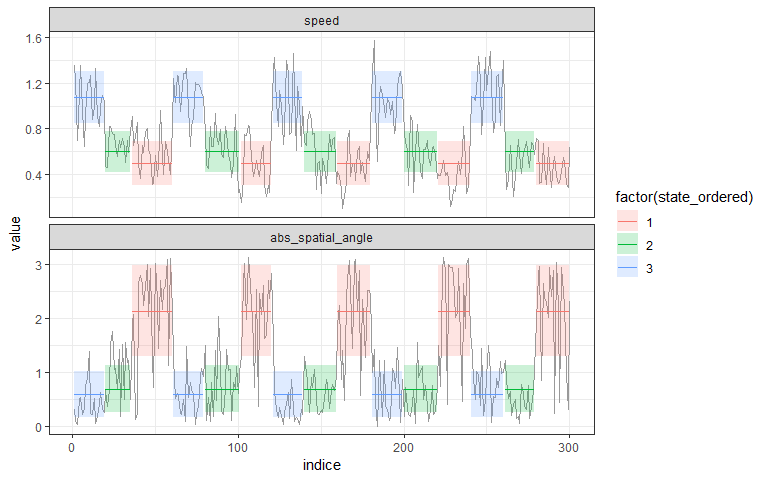By default for a segmentation/clustering, plot.segmentation shows the best segmentation, maximizing BIC-based penalized likelihood, but one can specify a given number of cluster/or segment See segmentation-class for additional informations.

plot(mode_segclust, ncluster = 3)
#> User-specified number of class :3
#> BIC-selected number of segment : 15
plot(mode_segclust, ncluster = 3, nseg = 7)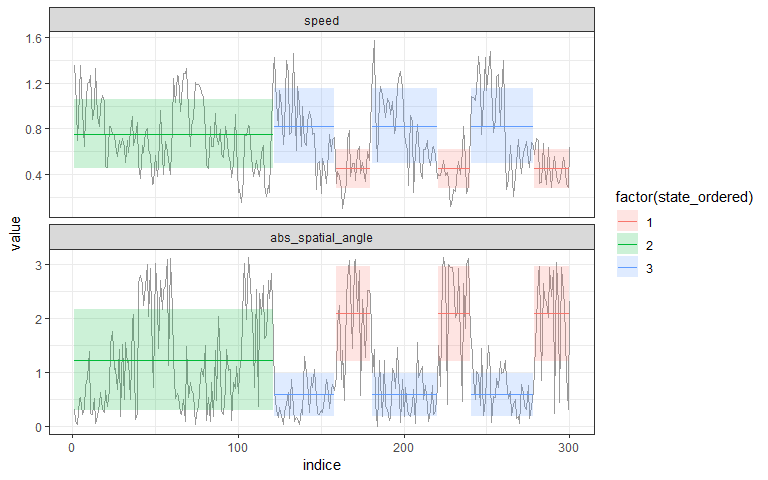One can also inspect the BIC-based penalized log-likelihood through functions plot_BIC(). Best-case scenario is as below, the BIC show a steep increase up to a maximum and a slow decrease after the optimum and one number of cluster is clearly above the others. With real data it also happens that more cluster always improve the penalized-likelihood but so we generally advise to choose the number of cluster based on expectation and biological knowledge.

plot_BIC(mode_segclust)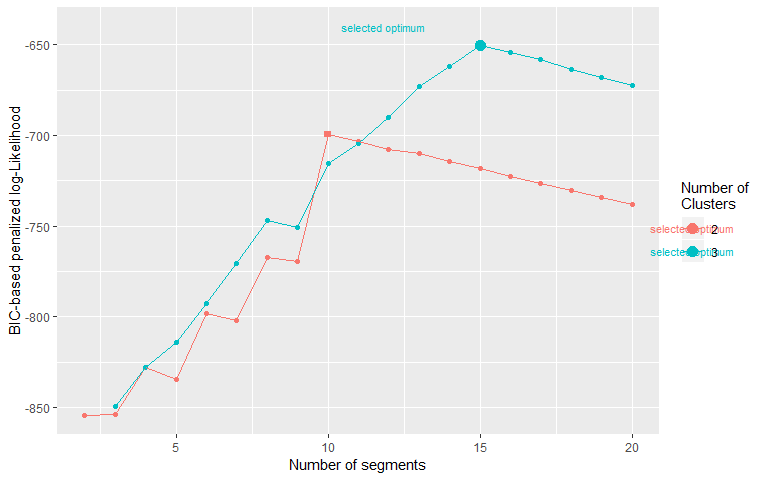## Advice for choosing lmin, Kmax and ncluster

lmin is the minimum length of a segment. For home range it is the duration for which we consider a stationary use to be a home-range. For behaviour it is the minimum time for a behaviour not te be considered anecdotical.

Kmax is by default fixed to the maximum but for performance we advise on setting a smaller Kmax. If the selected number of segment is too close to Kmax, think about increasing Kmax, that might be limiting the number of segment. As noted before, when Kmax is to close to the maximum (n/lmin) the algorithm may oversegment and we advise to look carefully at the likelihood curve when using Kmax > 0.75*n/lmin

By default ncluster is chosen by maximizing a BIC-based penalized log-likelihood. When segmentation-clustering is reliable, the selected optimum should the maximum just before a linear drop of the penalized log-Likelihood. Also even though higher number of cluster may have higher penalized log-Likelihood the difference between them should not be too large. Also, as in this example, if the selected number of segment for a higher number of cluster is the same, then the lower number should be preferred. Not that this selection of number of cluster is mostly a suggestion and should not be trusted. Best practice should rely on biological information to fix a priori the number of states.

# Exploring outputs

Both functions segmentation() and segclust() returns a segmentation-class object for which several methods are available.

## Extract information about predicted states.

### augment - get state for each point.

augment.segmentation() is a method for broom::augment. It returns an augmented data.frame with outputs of the model - here, the attribution to segment or cluster

augment(shift_seg)
#> Lavielle-selected number of segment : 3
augment(mode_segclust)
#> BIC-selected number of class : 3 class.
#> BIC-selected number of segment : 15

By default augment.segmentation will use data for the best segmentation (maximum of penalized log-Likelihood for segclust() and Lavielle’s criterium for segmentation()) but one can ask for a specific segmentation :

augment(shift_seg, nseg = 10) # segmentation()
augment(mode_segclust, ncluster = 2) # segclust()
#> User-specified number of class :2
#> BIC-selected number of segment : 10
augment(mode_segclust, ncluster = 2, nseg = 5) # segclust()

### segment - Extract each segment (begin, end, statistics)

segment() allows retrieving informations on the different segment of a given segmentation. Each segment is associated with the mean and standard deviation for each variable, the state (equivalent to the segment number for segmentation) and the state ordered given a variable - by default the first variable given by seg.var. One can specify the variable for ordering states through the order.var of segmentation() and segclust().

segment(shift_seg)
#> Lavielle-selected number of segment : 3
segment(shift_seg, nseg = 3)
segment(mode_segclust)
#> BIC-selected number of class : 3 class.
#> BIC-selected number of segment : 15
segment(mode_segclust, nclust = 3, nseg = 8)

### states - statistics about each states.

states() return information on the different states of the segmentation. For segmentation() it is quite similar to segment(). For segclust, however it gives the different cluster found and the statistics associated.

states(shift_seg)
#> Lavielle-selected number of segment : 3
states(shift_seg, nseg = 3)
states(mode_segclust)
#> BIC-selected number of class : 3 class.
#> BIC-selected number of segment : 15
states(mode_segclust, nclust = 3, nseg = 8)

### log-Likelihood - logLik

logLik.segmentation() return information on the log-likelihood of the different segmentations possible. It returns a data.frame with the number of segment, the log-likelihood and eventually the number of cluster.

logLik(shift_seg)
logLik(mode_segclust)

### BIC-based penalized likelihood (segclust)

BIC.segmentation() return information on the BIC-based penalized log-likelihood of the different segmentations possible. It returns a data.frame with the number of segment, the BIC-based penalized log-likelihood and the number of cluster. For segclust() only. Note that this does not truly returns a BIC.

BIC(mode_segclust)

## Graphical outputs

segmentation-class also provides methods for plotting results of segmentations. All plot methods use ggplot2 package and return ggplot objects that can be further modified and customized using classical ggplot2 (see ggplot2 function reference.

### plot.segmentation - series plot of the segmentation

plot.segmentation() can be used to plot the output of a segmentation as a series-plot. A specific segmentation can be chosen with nseg and ncluster arguments. If the original data had a specific x-axis, like a POSIXct time column, this can be specified using argument xcol. By default, data are plotted by their number. If you want clusters or segments to be ordered according to one of the variables, this can be specified using argument order. By default segmentation/clustering output are plotted using ordered states.

plot(shift_seg)
#> Lavielle-selected number of segment : 3
plot(mode_segclust, ncluster = 3, nseg = 10, xcol = "indice", order = T)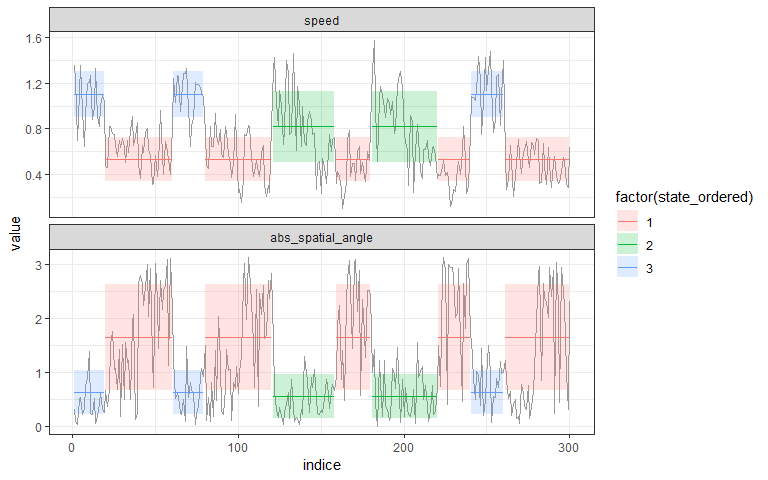Here there was a fake dateTime column in POSIXct format in the data.frame originally given to the function segclust. We can then plot the results of the segmentation according the the time given.

plot(mode_segclust, ncluster = 3, nseg = 10, xcol = "dateTime", order = T)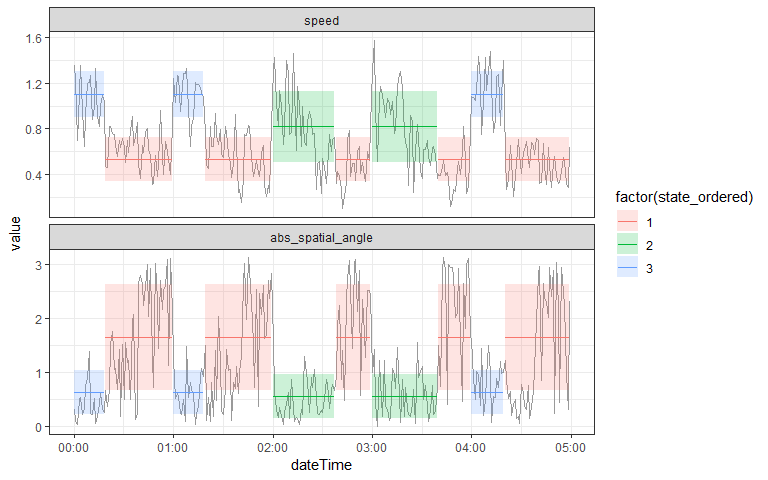### segmap - map the segmentation

segmap() plot the results of the segmentation as a map. This can be done only if data have a geographic meaning. Coordinate names are by default “x” and “y” but this can be provided through argument coord.names.

segmap(shift_seg, nseg = 10)
segmap(mode_segclust, ncluster = 3, nseg = 10)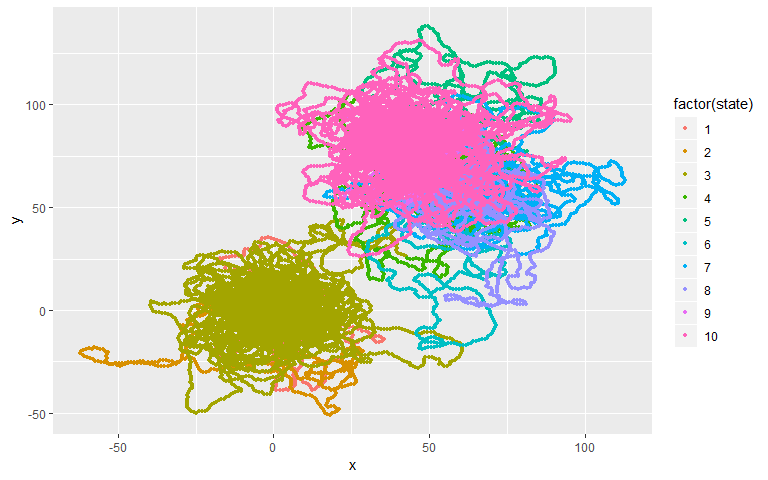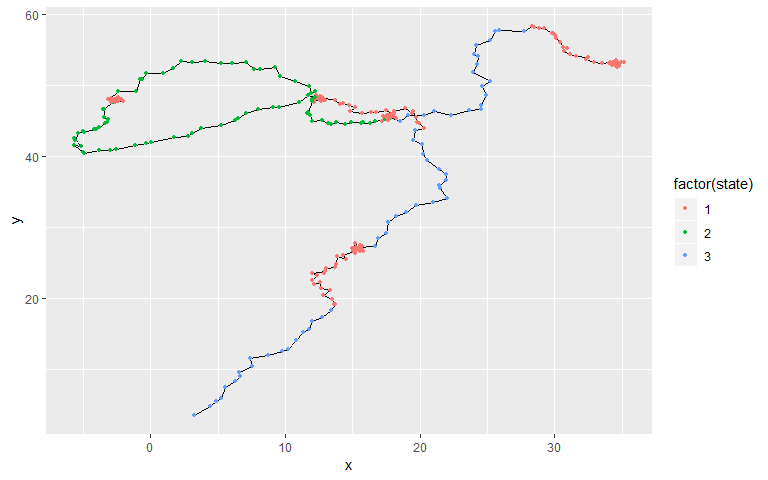### stateplot - plot states statistics

stateplot() show statistics for each state or segment.

stateplot(shift_seg, nseg = 10)
stateplot(mode_segclust, ncluster = 3, nseg = 10)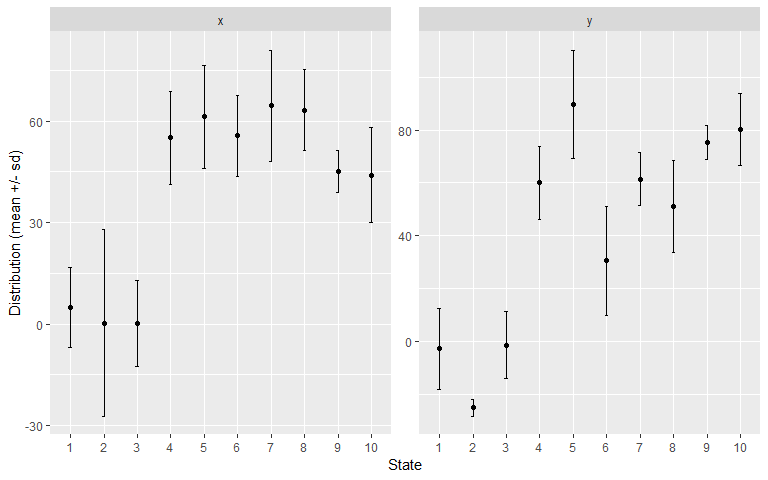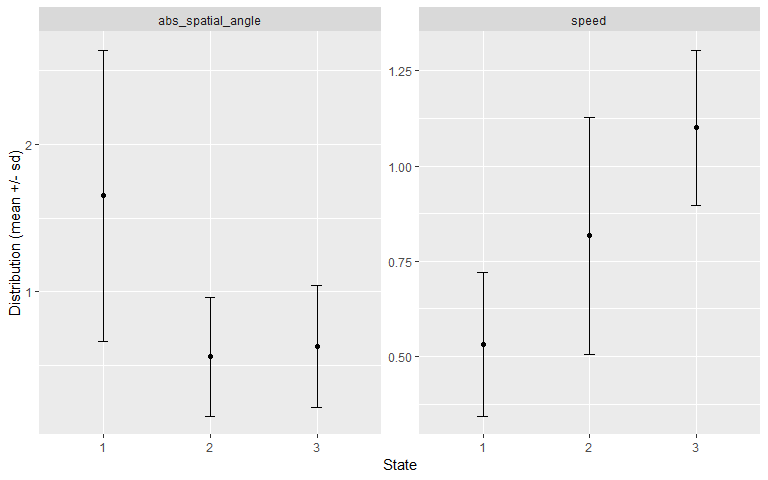### plot_likelihood

plot_likelihood() plot the log-likelihood of the segmentation for all the tested number of segments and clusters.

plot_likelihood(shift_seg)
plot_likelihood(mode_segclust)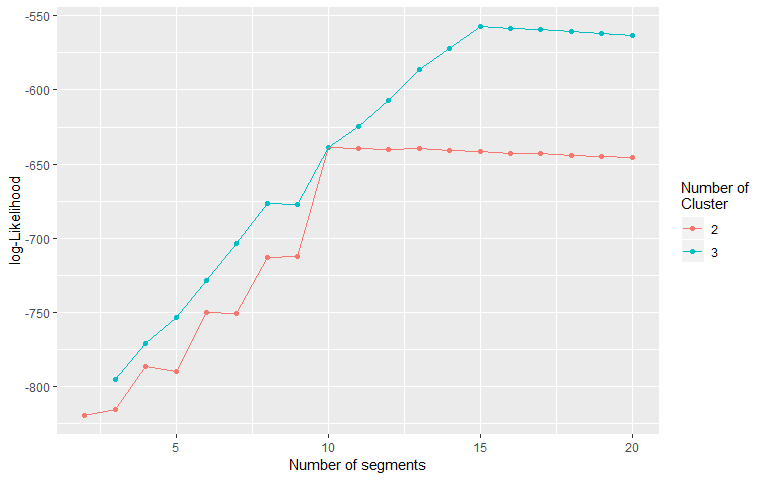### plot_BIC - plot the BIC-based penalized likelihood

plot_BIC() plot the BIC-based penalized log-likelihood of the segmentation for all the tested number of segments and clusters.

plot_BIC(mode_segclust)# Advanced Options

## Subsampling

Computation cost for the algorithm scales non-linearly and can be both memory and time-consuming. Performance depends on computer, but from what we’ve tested, a segmentation on data of size > 10000 can be quite memory consuming (more than 10Go of RAM) and segmentation-clustering can be quite long for data > 1000 (few minutes to hours). For such dataset we recommand either subsampling if loosing resolution is not a big deal (looking for home-range changes over a year with hourly points might be a lost of time when daily points are sufficient) or if splitting the dataset for very long data. Although for segmentation-clustering, clusters will not be easily comparable between the different part of the dataset, if one provides parts where all cluster are present for sure, there should be ne problem.

3 different options are available for subsampling. First one can disable subsampling through argument subsample :

mode_segclust <- segclust(simulmode, Kmax = 30, lmin=5, ncluster = c(2,3,4), type = "behavior", seg.var = c("speed","abs_spatial_angle"), subsample = FALSE)

By default subsampling is allowed (subsample = TRUE) and subsampling will occur if the number of data exceed a threshold (10000 for segmentation, 1000 for segmentation-clustering). The function will subsample by the lower factor (by 2, 3, 4…) for which the dataset will fall below the threshold once subsampled. For instance a 2500 rows dataset for segmentation-clustering would be subsampled by 3 to fall below 1000 rows. The threshold can be changed through argument subsample_over.

mode_segclust <- segclust(simulmode, Kmax = 30, lmin=5, ncluster = c(2,3,4), type = "behavior", seg.var = c("speed","abs_spatial_angle"), subsample_over = 1000)

One can also override this automatic subsampling by selecting directly the subsampling factor through argument subsample_by.

mode_segclust <- segclust(simulmode, Kmax = 30, lmin=5, ncluster = c(2,3,4), type = "behavior", seg.var = c("speed","abs_spatial_angle"), subsample_by = 2)

Beware that subsampling will also divide your lmin argument. If subsampling by 2, lmin will be divided by 2. It is important that lmin stays larger than 3 and if possible than 5, for better variance estimations.

Note that subsampling has been implemented in such way that outputs will show all points but segmentation is calculated only on subsampled points. Points used in segmentation can be retrieved through augment in data column subsample_ind (The subsample indices for kept points and NA for ignored points).

## Other data types

We have shown examples for using data.frames but one can also segment data from ltraj and Move object that contains a single individual.

### Concerning segmentation

For a simple segmentation, the algorithm will assume a home-range segmentation and use coordinates directly.

segmentation(ltraj_object, lmin = 5, Kmax = 25)
segmentation(Move_object, lmin = 5, Kmax = 25)

### Concerning segclust

For a segmentation/clustering, one has to provide the variables used for segmentation

segmentation(ltraj_object, lmin = 5, Kmax = 25, ncluster = c(2,3), seg.var = c("speed","abs_spatial_angle"))
segmentation(Move_object, lmin = 5, Kmax = 25, ncluster = c(2,3), seg.var = c("speed","abs_spatial_angle"))

Of course the variable names provided must exist as column in Move_object@data and adehabitatLT::infolocs(ltraj_object).

# Covariate calculations

The package also includes functions in order to calculate unusual covariates, such as the turning angle at constant step length (here called spatial_angle). For the latter, a radius have to be chosen and can be specified through argument radius. If no radius is specified, the default one will be the median of the step length distribution. Other covariates calculated are : peristence and turning speed (v_p and v_r) from Gurarie et al (2009), distance travelled between points, speed and smoothed version of the latter. Covariates dependent on time interval (like speed) are by default calculated with hours, but you can change this with argument units as in the example below.

simple_data <- simulmode[,c("dateTime","x","y")]
full_data   <- add_covariates(simple_data, coord.names = c("x","y"), timecol = "dateTime",smoothed = TRUE, units ="min")
head(full_data)